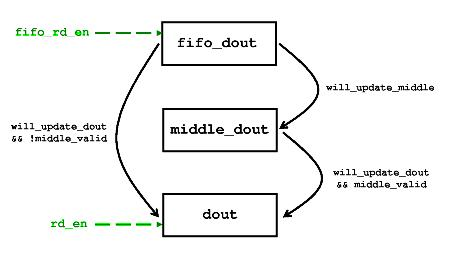01signal.com

# 通过添加 registers在 FIFOs 上改进 timing

### Standard FIFO 至 FWFT FIFO

@rd_en 的含义也不同： 对于“standard FIFO”，它的意思是“给我带来数据”。在 FWFT FIFO 上，它类似于“我刚刚使用了数据，如果你有下一个数据，请给我带来”。

```module basic_fwft_fifo(rst,
rd_clk, rd_en, dout, empty,
wr_clk, wr_en, din, full);

parameter width = 8;

input                 rst;
input                 rd_clk;
input                 rd_en;
input                 wr_clk;
input                 wr_en;
input [(width-1):0]   din;
output                empty;
output                full;
output [(width-1):0]  dout;

reg                   dout_valid;
wire                  fifo_rd_en, fifo_empty;

// orig_fifo is just a normal (non-FWFT) synchronous or asynchronous FIFO
fifo orig_fifo
(
.rst(rst),
.rd_clk(rd_clk),
.rd_en(fifo_rd_en),
.dout(dout),
.empty(fifo_empty),
.wr_clk(wr_clk),
.wr_en(wr_en),
.din(din),
.full(full)
);

assign fifo_rd_en = !fifo_empty && (!dout_valid || rd_en);
assign empty = !dout_valid;

always @(posedge rd_clk or posedge rst)
if (rst)
dout_valid <= 0;
else
begin
if (fifo_rd_en)
dout_valid <= 1;
else if (rd_en)
dout_valid <= 0;
end
endmodule```

### FWFT FIFO 至 standard FIFO

```module standard_fifo(rst,
rd_clk, rd_en, dout, empty,
wr_clk, wr_en, din, full);

parameter width = 8;

input                 rst;
input                 rd_clk;
input                 rd_en;
input                 wr_clk;
input                 wr_en;
input [(width-1):0]   din;
output                empty;
output                full;
output [(width-1):0]  dout;

reg [(width-1):0]     dout;
wire [(width-1):0]    dout_w;

always @(posedge rd_clk)
if (rd_en && !empty)
dout <= dout_w;

fwft_fifo wrapper
(
.wr_clk(wr_clk),
.rd_clk(rd_clk),
.rst(rst),
.din(din),
.wr_en(wr_en),
.rd_en(rd_en && !empty),
.dout(dout_w),
.full(full),
.empty(empty)
);
endmodule```

### 当 @empty 和/或 @full 在 critical path中时

@empty 信号和 @full 信号可能出现在 critical path中，特别是如果 @wr_en 和 @rd_en 是其中的 combinatorial functions 。这主要是因为这些信号通常不仅用于请求来自 FIFO 的写入操作或读取操作，而且它们还充当 enable signals 的 enable signals 用于消费或产生数据的 application logic ： 如果数据没有流动， logic 也会冻结。

### 当 @wr_en 和/或 @din 在 critical path中时

```always @(posedge wr_clk)
begin
wr_en_reg <= wr_en;
din_reg <= din_reg;
end```

### 当 @rd_en 和/或 @dout 在 critical path中时

• @rd_en 信号在 FIFO内部的 combinatorial logic 中使用，除此之外，在计算下一个 read address时。所以 FIFO 本身贡献了 delay的数量。
• 生成 @rd_en 的 application logic 通常是可以复杂的 combinatorial function 。这个 logic function 可能由 state registers 和来自 logic fabric不同部分的几个 flags 组成。

@dout：

• FIFO的 data output 通常直接连接到 block RAM。与 FPGA的 flip-flop相比，这些 RAMs 的 clock-to-output timing明显更差。如果 FIFO 是用几个 RAMs实现的，它们的 data outputs 被插入到一个 multiplexer中，所以 @dout 就是这个 combinatorial logic的结果。这增加了更多的 delay。
• application logic 可以使用 combinatorial functions中 @dout 的值。
• FIFO的 RAMs 和 application logic 在 FPGA 上的物理距离可以在大 FPGAs上加上 delay 。

### 将 combinatorial paths 与 reg_fifo分离

```module reg_fifo(rst,
rd_clk, rd_en, dout, empty,
wr_clk, wr_en, din, full);

parameter width = 8;

input                 rst;
input                 rd_clk;
input                 rd_en;
input                 wr_clk;
input                 wr_en;
input [(width-1):0]   din;
output                empty;
output                full;
output [(width-1):0]  dout;

reg                   fifo_valid, middle_valid;
reg [(width-1):0]     dout, middle_dout;

wire [(width-1):0]    fifo_dout;
wire                  fifo_empty, fifo_rd_en;
wire                  will_update_middle, will_update_dout;

// orig_fifo is "standard" (non-FWFT) FIFO
fifo orig_fifo
(
.rst(rst),
.rd_clk(rd_clk),
.rd_en(fifo_rd_en),
.dout(fifo_dout),
.empty(fifo_empty),
.wr_clk(wr_clk),
.wr_en(wr_en),
.din(din),
.full(full)
);

assign will_update_middle = fifo_valid && (middle_valid == will_update_dout);
assign will_update_dout = rd_en && !empty;
assign fifo_rd_en = !fifo_empty && !(middle_valid && fifo_valid);
assign empty = !(fifo_valid || middle_valid);

always @(posedge rd_clk)
if (rst)
begin
fifo_valid <= 0;
middle_valid <= 0;
dout <= 0;
middle_dout <= 0;
end
else
begin
if (will_update_middle)
middle_dout <= fifo_dout;

if (will_update_dout)
dout <= middle_valid ? middle_dout : fifo_dout;

if (fifo_rd_en)
fifo_valid <= 1;
else if (will_update_middle || will_update_dout)
fifo_valid <= 0;

if (will_update_middle)
middle_valid <= 1;
else if (will_update_dout)
middle_valid <= 0;
end
endmodule```

### 了解 pipeline`assign empty = !(fifo_valid || middle_valid);`

`assign fifo_rd_en = !fifo_empty && !(middle_valid && fifo_valid);`

```assign will_update_middle = fifo_valid && (middle_valid == will_update_dout);
assign will_update_dout = rd_en && !empty;```

```always @(posedge rd_clk)
if (will_update_middle)
middle_dout <= fifo_dout;```

• @middle_valid == 0 和 @will_update_dout == 0。 @middle_dout 无效， @fifo_dout的值不会被复制到 @dout中。所以从 @fifo_dout更新 @middle_dout 。
• @middle_valid == 0 和 @will_update_dout == 1。 @middle_dout 是无效的，但是 @dout 会更新，所以显然它会从 @fifo_dout更新。因为 @fifo_dout的值被消耗了，所以不能复制到 @middle_out中，所以什么也不做。
• @middle_valid == 1 和 @will_update_dout == 0。 @middle_dout 是有效的，没有任何东西被复制到 @dout中。两个 pipeline stages 都是有效的，并且无论如何都将保持有效。所以什么也不做。
• @middle_valid == 1 和 @will_update_dout == 1。 @middle_dout 有效，将被复制到 @dout中。因此，从 @fifo_dout 更新 @middle_dout 以保持有效。

```always @(posedge rd_clk)
if (will_update_dout)
dout <= middle_valid ? middle_dout : fifo_dout;```

```always @(posedge rd_clk)
if (rd_en && !empty)
dout <= middle_valid ? middle_dout : fifo_dout;```

### 跟踪 pipeline stages的 registers的有效性

```if (fifo_rd_en)
fifo_valid <= 1;
else if (will_update_middle || will_update_dout)
fifo_valid <= 0;```

@middle_valid 遵循相同的逻辑：

```if (will_update_middle)
middle_valid <= 1;
else if (will_update_dout)
middle_valid <= 0;```

### 它真的有效吗？

```wire [1:0] valid_count;
assign valid_count = fifo_valid + middle_valid;```

• @valid_count 在 @fifo_rd_en 为且 (rd_en && !empty) 为false的每个 clock cycle 上加一。
• @valid_count 在 @fifo_rd_en 为且 (rd_en && !empty) 为true的每个 clock cycle 上减一。
• 否则， @valid_count 不会改变。

reg_fifo的 logic 试图通过读取 orig_fifo将 @valid_count 推向 2。另一方面，当 @valid_count 不为零时， @empty 为低电平，因此一旦 @valid_count 为 1， @rd_en 就被允许变高。因此，当 @valid_count 为 1 时， @fifo_rd_en 将为高电平，因为 @valid_count 不是 2。

### 使用说明

reg_fifo 的一个小缺点是它的 @empty output 不是 register，而是两个 registers的 combinatorial function 。这不是 timing的最佳选择，但在大多数用例中影响很小。这可以通过以与 @next_words_in_ram相同的精神定义 combinatorial registers 来解决，如 @next_fifo_valid 和 @next_middle_valid ，如本页所示。这里没有实现，主要是因为 reg_fifo 已经足够复杂了。

### 带有改进的 timing的 FWFT FIFO

```module fwft_reg_fifo(rst,
rd_clk, rd_en, dout, empty,
wr_clk, wr_en, din, full);

parameter width = 8;

input                 rst;
input                 rd_clk;
input                 rd_en;
input                 wr_clk;
input                 wr_en;
input [(width-1):0]   din;
output                empty;
output                full;
output [(width-1):0]  dout;

reg                   middle_valid, dout_valid;
reg [(width-1):0]     dout, middle_dout;

wire [(width-1):0]    fifo_dout;
wire                  fifo_empty, fifo_rd_en;
wire                  will_update_middle, will_update_dout;

// orig_fifo is "standard" (non-FWFT) FIFO
fifo orig_fifo
(
.rst(rst),
.rd_clk(rd_clk),
.rd_en(fifo_rd_en),
.dout(fifo_dout),
.empty(fifo_empty),
.wr_clk(wr_clk),
.wr_en(wr_en),
.din(din),
.full(full)
);

assign will_update_middle = !fifo_empty && (middle_valid == will_update_dout);
assign will_update_dout = (middle_valid || !fifo_empty) && (rd_en || !dout_valid);
assign fifo_rd_en = !fifo_empty && !(middle_valid && dout_valid);
assign empty = !dout_valid;

always @(posedge rd_clk)
if (rst)
begin
middle_valid <= 0;
dout_valid <= 0;
dout <= 0;
middle_dout <= 0;
end
else
begin
if (will_update_middle)
middle_dout <= fifo_dout;

if (will_update_dout)
dout <= middle_valid ? middle_dout : fifo_dout;

if (will_update_middle)
middle_valid <= 1;
else if (will_update_dout)
middle_valid <= 0;

if (will_update_dout)
dout_valid <= 1;
else if (rd_en)
dout_valid <= 0;
end
endmodule```